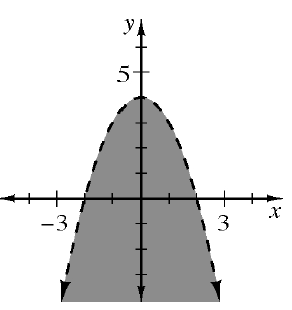### Home > GC > Chapter 10 > Lesson 10.2.1 > Problem10-62

10-62.

A graph of an inequality is shown at right. Decide if each of the points $\left(x,y\right)$ listed below would make the inequality true or not. For each point, explain how you know.

If a point makes the inequality true, it must be in the shaded region.1. $\left(1,1\right)$

True

1. $\left(-3,2\right)$

False

1. $\left(-2,0\right)$

False

1. $\left(0,-2\right)$

True